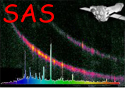XMM-Newton Science Analysis System

psfgen (psfgen-1.7) [xmmsas_20211130_0941-20.0.0]

## Example 5: Coordinates from an input image

A PSF for a particular source in a sky-coordinate image can be produced by supplying the name of the image and the position in sky (XY) or celestial (RA,DEC) or image pxiel coordinates. e.g.

psfgen image=myskyimage.ds energy=3000 level=ELLBETA coordtype=POS x=27540 y=15620 output=psf.fits

or

psfgen image=myskyimage.ds energy=3000 level=ELLBETA coordtype=EQPOS x=221.7612 y=68.8493
xsize=400 ysize=400 output=psf.fits

psfgen image=myskyimage.ds energy=3000 level=EXTENDED coordtype=IMPIX x=239 y=186 output=psf.fits

where the first comand produces a 199 x 199 pixel (default size) PSF image centred on the sky coordinate (XY) position (27540,15620), the second produces a 400x400 pixel image centred on the celestial position, RA: 221.7612, DEC: 68.8493 and the third produces an image centred on the position of the input image pixel (239,186). The task uses the world coordinate system information encoded in the header of the input image to identify the location of the PSF centre and uses the position angle of the observation (keyword=PA_PNT) to rotate the output image appropriately. Naturally, these values have to be correctly set in the input image, which they should be if they have been created by the SAS tasks evselect or xmmselect.

The PSF is made for the camera contained in the INSTRUME keyword in the header of the file myskyimage.ds. This may be overridden by setting the instrument paremeter directly. e.g.

psfgen image=myskyimage.ds energy=3000 level=EXTENDED coordtype=IMPIX x=239 y=186 instrument=EPN

XMM-Newton SOC -- 2021-11-30